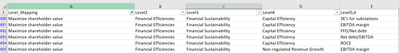cancel
Showing results for
Did you mean:Helper IV

## Average for Hierarchial Data not working as Expected

Hi ,

I have the following requirement where i need to show performance of the hierarchial data across level 1,2,3,4,5.

So average performance of level 4 = Average of level 5, average performance of level 3 = average of level 4 and so on.

We have hierarchial data already in our model as below.

The issue is , we are averaginf the performance and if you consider below example , as per hierarchy , sum of average performance of level 4 is performance of level 3 .

For below financial sustainability for level 3 overall performance is 54% which should be average of level 4 ( 50%,44%,55% ,74%) but if we calculate average its actually 56%.

I have already tried average by category in quick measure as below which is also not working as expected.

Performance_New average per Item =
AVERAGEX(
KEEPFILTERS(VALUES('All_Bls_Fct'[Item])),
CALCULATE(SUM(All_Bls_Fct[Performance_New])
))

and also tried below measure.

`AVERAGEX(VALUES(Table4[Product]),CALCULATE(SUM([Amount])))`

Note: Since hierarchty is already available in our model i have just created single measure for Performance and using it across and i am using average option from power bi default option.

Kindly help on how to proceed with this issue. Thanks in advance.4 REPLIES 4Super User

If you want level 4 to averaged at level 3

Performance_New average per Item =
AVERAGEX(
(VALUES('Table'[Level 4]),
CALCULATE(SUM(All_Bls_Fct[Performance_New])
))Helper IV

Hi @amitchandak This is not working as expected as we have data only for level 5,6 , all these 5 and 6 have to roll up till level 1 , hence cannot separately calculate average for level 4 separately.Super User

@binayjethwa , Using isinscope measure for each level

Switch(True() ,

isinscope(Table[Level 6]) , SUM(All_Bls_Fct[Performance_New]) , //lowest level

isinscope(Table[Level 5]) , AVERAGEX(VALUES('Table'[Level 6]),CALCULATE(SUM(All_Bls_Fct[Performance_New]))),

isinscope(Table[Level 4]) , AVERAGEX(VALUES('Table'[Level 5]),CALCULATE(SUM(All_Bls_Fct[Performance_New]))),

)Helper IV

Hi @amitchandak

This will be my hierarchial data and i have mapped it to my fact table with level 5,6 which are common across 2 tables.

I can calculate performance only based on values givrn for level 5,6. Hence how do i create measure which will give these averages correctly. Level_mapping is level .Announcements#### Exclusive opportunity for Women!

Join us for a free, hands-on Microsoft workshop led by women trainers for women where you will learn how to build a Dashboard in a Day!#### Power Platform Conference-Power BI and Fabric Sessions

Join us Oct 1 - 6 in Las Vegas for the Microsoft Power Platform Conference.Top Solution Authors
Top Kudoed Authors
Users online (3,690)